### THE METHOD OF INTEGRATION BY POWER SUBSTITUTION

The following problems involve the method of power substitution. It is a method for finding antiderivatives of functions which contain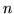th roots ofor other expressions. Examples of such expressions areand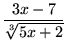.

The method of power substitution assumes that you are familiar with the method of ordinary u-substitution and the use of differential notation. Recall that if,

then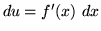.

For example, if,

then.

When using the method of power substitution, we will usually assume that,

so that.

For example, if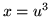,

then.

The goal of a power substitution will be to replaceth roots of functions, which may not be easily integrated, with integer powers of functions, which are more easily integrated. For example, if we start with the expressionand let,

thenand.

Thus,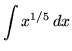could be rewritten as.

In general, to eliminate,

let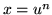.

Thenand.

Recall the following well-known, basic indefinite integral formulas :

• 1.), where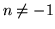• 2.)• 3.)• 4.)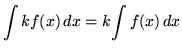, whereis a constant
• 5.)We can now apply the method of power substitution to an integral like.

A COMMON WRONG ANSWER FOR THIS PROBLEM IS.

Convince yourself that the answer is wrong by differentiating the right-hand side and showing it is NOT equal to the function on the left-hand side of the above equation. To properly integrate this problem, use the power substitutionso thatand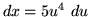.

Now substitute into the original problem, replacing all forms of, getting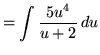(Use polynomial division.)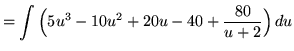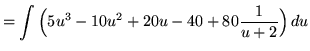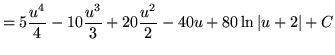.

Most of the following problems are average. A few are challenging. Make careful and precise use of the differential notationandand be careful when arithmetically and algebraically simplifying expressions. You will likely need to use the following rules for exponents.

•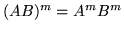•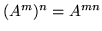••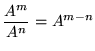• PROBLEM 1 : Integrate.

• PROBLEM 2 : Integrate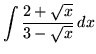.

• PROBLEM 3 : Integrate.

• PROBLEM 4 : Integrate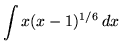.

• PROBLEM 5 : Integrate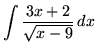.

• PROBLEM 6 : Integrate.

• PROBLEM 7 : Integrate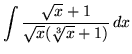.

• PROBLEM 8 : Integrate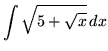.

• PROBLEM 9 : Integrate.

• PROBLEM 10 : Integrate.

• PROBLEM 11 : Integrate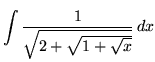.

• PROBLEM 12 : Integrate.

• PROBLEM 13 : Integrate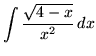.

• PROBLEM 14 : Integrate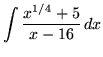.